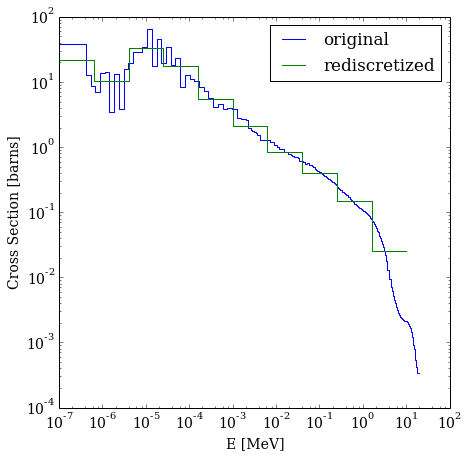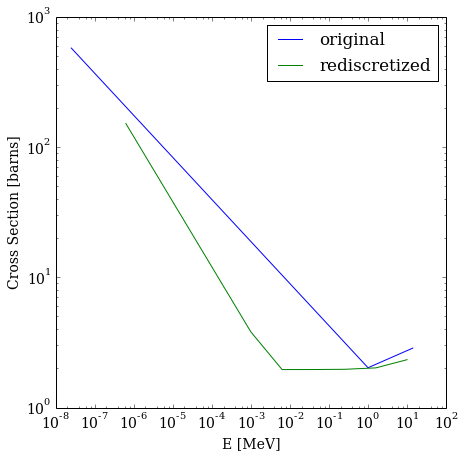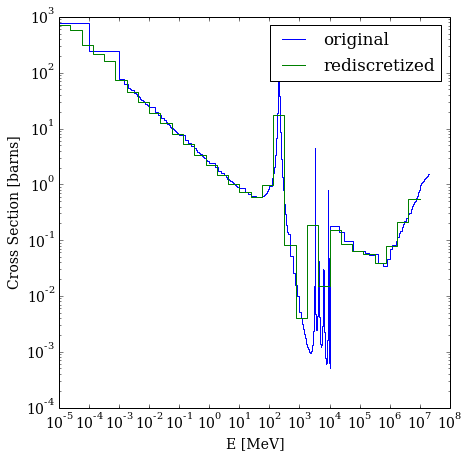# Cross Sections¶

Download the full notebook.

05-cross-sections

# Cross Section Interface¶

PyNE provides a top-level interface for computing (and caching) multigroup neutron cross sections. These cross sections will be computed from a variety of available data sources (stored in nuc_data.h5). This interface remains the same across all data sources, allowing the user to easily swap out which cross section library they wish to use.

The following cross section formats are included with PyNE.

• The ENDF file format (experimental),
• The ACE file format,
• EAF 175-group cross sections,
• CINDER 63-group cross sections,
• A two-point fast/thermal interpolation (using 'simple_xs' data from KAERI),
• Physical models, and
• Null data.

This functionality may be be found in the xs sub-package. This package is separated out into the following modules:

models
data_source
cache
channels

## Data Sources¶

Data sources are classes that provide the common interface for grabbing cross section data from a data source. Unless otherwise specified, this is lazily evaluated. The data source object itself then acts as an in-memory cache, making further look ups of the raw data very quick!

To get a sense of how this works, let's pull in some data that we know about.

### EAF Data Source¶

In :
from pyne.xs.data_source import EAFDataSource, SimpleDataSource, NullDataSource

In :
eds = EAFDataSource()
# reaction returns a dictionary containing source group structure, energy values,
# cross-section data, and interpolation data.
gamma = eds.reaction('U235', 'gamma')
gamma

Out:
array([  3.40601000e-04,   4.13527000e-04,   4.52339000e-04,
5.35260000e-04,   7.79656000e-04,   9.12824000e-04,
1.05027000e-03,   1.20057000e-03,   1.33202000e-03,
1.46200000e-03,   1.60595000e-03,   1.67206000e-03,
1.76457000e-03,   1.90211000e-03,   2.03703000e-03,
2.12614000e-03,   2.15500000e-03,   2.15923000e-03,
2.17015000e-03,   2.19708000e-03,   2.24992000e-03,
2.34009000e-03,   2.47761000e-03,   2.65621000e-03,
2.80624000e-03,   2.93429000e-03,   3.18937000e-03,
3.54963000e-03,   3.99022000e-03,   4.53866000e-03,
5.21324000e-03,   6.05776000e-03,   7.06523000e-03,
9.26177000e-03,   1.27481000e-02,   1.75162000e-02,
2.23344000e-02,   2.54474000e-02,   2.88533000e-02,
3.21686000e-02,   3.59024000e-02,   4.03127000e-02,
4.31805000e-02,   4.57085000e-02,   4.57084000e-02,
4.57112000e-02,   4.87787000e-02,   5.18315000e-02,
5.62859000e-02,   6.08943000e-02,   6.55405000e-02,
7.01358000e-02,   7.41851000e-02,   7.78783000e-02,
8.13216000e-02,   8.57563000e-02,   8.88694000e-02,
9.13386000e-02,   9.53971000e-02,   9.82263000e-02,
1.00158000e-01,   1.04962000e-01,   1.08964000e-01,
1.11877000e-01,   1.14201000e-01,   1.16384000e-01,
1.19622000e-01,   1.23457000e-01,   1.27270000e-01,
1.31726000e-01,   1.35999000e-01,   1.40273000e-01,
1.44433000e-01,   1.49346000e-01,   1.55223000e-01,
1.60902000e-01,   1.69406000e-01,   1.83582000e-01,
1.92772000e-01,   1.99107000e-01,   2.07917000e-01,
2.18980000e-01,   2.24834000e-01,   2.28807000e-01,
2.28834000e-01,   2.28851000e-01,   2.32272000e-01,
2.47665000e-01,   2.60721000e-01,   2.69491000e-01,
2.77787000e-01,   2.86525000e-01,   2.95251000e-01,
3.03786000e-01,   3.12412000e-01,   3.20788000e-01,
3.28606000e-01,   3.35689000e-01,   3.45583000e-01,
3.55016000e-01,   3.64635000e-01,   3.74740000e-01,
3.83871000e-01,   3.96374000e-01,   4.20446000e-01,
4.36756000e-01,   4.49877000e-01,   4.66706000e-01,
4.97313000e-01,   5.05926000e-01,   5.32346000e-01,
5.64841000e-01,   5.50892000e-01,   5.98351000e-01,
6.17782000e-01,   6.72248000e-01,   6.96237000e-01,
6.99460000e-01,   7.01140000e-01,   7.19296000e-01,
7.33180000e-01,   7.24884000e-01,   7.69404000e-01,
7.77250000e-01,   8.32799000e-01,   9.33925000e-01,
1.01020000e+00,   1.07310000e+00,   1.21651000e+00,
1.27445000e+00,   1.30837000e+00,   1.52976000e+00,
1.62957000e+00,   1.69051000e+00,   1.74094000e+00,
1.83856000e+00,   1.95794000e+00,   1.97871000e+00,
2.60955000e+00,   2.70133000e+00,   2.82330000e+00,
3.85308000e+00,   4.03064000e+00,   3.91338000e+00,
4.59505000e+00,   4.20223000e+00,   5.61362000e+00,
7.39548000e+00,   8.33983000e+00,   1.02707000e+01,
1.11389000e+01,   1.29232000e+01,   8.42540000e+00,
2.31086000e+01,   1.80067000e+01,   3.46571000e+01,
1.94390000e+01,   4.63772000e+01,   1.76541000e+01,
6.54149000e+01,   3.43397000e+01,   2.86602000e+01,
2.89308000e+01,   1.97519000e+01,   1.58082000e+01,
3.79141000e+00,   1.33372000e+01,   3.45039000e+00,
1.42551000e+01,   1.37460000e+01,   7.05691000e+00,
8.53781000e+00,   1.27605000e+01,   3.89028000e+01,
9.05356000e+01])

The data source also provides a method to re-discretize this data to another group structure.

In :
import numpy as np
dst_e_g = np.logspace(1, -7, 11)

# dst_group_struct is the group structure of the destination cross sections.
eds.dst_group_struct = dst_e_g

# discreatizes the reaction channel from the source group structure to that of the
# destination, weighted by the group fluxes.
gamma_c = eds.discretize('U235', 'gamma')
print(gamma_c)
print(dst_e_g)

[  2.52625773e-02   1.50668227e-01   3.94173552e-01   8.29069757e-01
2.08402584e+00   5.56199103e+00   1.73480167e+01   3.37415300e+01
1.01908466e+01   2.15494273e+01]
[  1.00000000e+01   1.58489319e+00   2.51188643e-01   3.98107171e-02
6.30957344e-03   1.00000000e-03   1.58489319e-04   2.51188643e-05
3.98107171e-06   6.30957344e-07   1.00000000e-07]


Now let's plot it!

In :
from pyne.bins import stair_step
%matplotlib inline
import matplotlib.pyplot as plt
import matplotlib
matplotlib.rc('font', family='serif', size=14)

In :
fig = plt.figure(figsize=(7,7))
plt.loglog(*stair_step(eds.src_group_struct, gamma), figure=fig) # original
plt.loglog(*stair_step(eds.dst_group_struct, gamma_c), figure=fig) # new group structure
plt.xlabel('E [MeV]')
plt.ylabel('Cross Section [barns]')
plt.legend(('original', 'rediscretized'))

Out:
<matplotlib.legend.Legend at 0x7f48e08631d0>### Simple Data Source¶

This interface is independent of where the data came from. As it should be!

In :
sds = SimpleDataSource(dst_group_struct=dst_e_g)
print(sds.exists)
rx = sds.reaction('U233', 'absorption') # cross section data
rxc = sds.discretize('U233', 'absorption') # discretization of data

True

In :
fig = plt.figure(figsize=(7,7))
plt.loglog(sds.src_group_struct[:-1], rx, figure=fig)
plt.loglog(sds.dst_group_struct[:-1], rxc, figure=fig)
plt.xlabel('E [MeV]')
plt.ylabel('Cross Section [barns]')
plt.legend(('original', 'rediscretized'))

Out:
<matplotlib.legend.Legend at 0x7f48e0b22210>Since data sources are caches, subsequent requests for the same data are very fast!

In :
%time sds.reaction('U238', 'fiss')
print
%time sds.reaction('U238', 'fission')
print
%time sds.reaction('U238', 'fiss')
print

CPU times: user 16.7 ms, sys: 1.32 ms, total: 18.1 ms
Wall time: 48.4 ms

CPU times: user 46 µs, sys: 7 µs, total: 53 µs
Wall time: 73 µs

CPU times: user 36 µs, sys: 7 µs, total: 43 µs
Wall time: 61 µs



## Cross Section Caching¶

Now suppose you want to perform the same discretization and wish to pull cross sections from many sources (with some precedence) based on whether the data is available or not. Enter XSCache. This is a class to do exactly that.

This class acts like a dictionary whose keys are (nuclide, rx) or (nuclide, rx, temp) tuples for the nuclide, reaction id, and temperature that you wish to know.

In :
from pyne.xs.cache import XSCache

In :
xscache = XSCache(group_struct=dst_e_g,
data_sources=[EAFDataSource, SimpleDataSource, NullDataSource])

In :
xscache['U235', 'abs']

Out:
array([   1.94057774,    1.71230641,    2.02444418,    3.24912017,
7.42318537,   20.15919429,   51.8621376 ,   77.11455565,
46.99610069,  155.69532222])
In :
xscache['H42', 'gamma']

Out:
array([ 0.,  0.,  0.,  0.,  0.,  0.,  0.,  0.,  0.,  0.])

## Reading ACE Files¶

To load data from an ACE file, one needs to simply initialize an instance of the Library class specifying the path to the Library file:

from pyne import ace
libFile = ace.Library('endf70a')
libFile.read()

## Reading ENDF Files¶

Similarly, ENDF file reading and cross-section discretization is supported.

In :
import requests
from pyne.xs.data_source import ENDFDataSource
from pyne import nucname
from os.path import isfile

# Download the data file if it isn't there
if not isfile("Ni59.txt"):
r = requests.get("http://t2.lanl.gov/nis/data/data/ENDFB-VII.1-neutron/Ni/59")
with open("Ni59.txt", "wb") as outfile:
outfile.write(r.content)

In :
endfds = ENDFDataSource("Ni59.txt")

# get the nonelastic reaction data for Ni-59
nonelastic_rx = endfds.reaction("Ni59", "nonelastic")

# set the group structure and re-discretize
nonelastic_rx['dst_group_struct'] = np.logspace(7, -5, 33)
nonelastic_c = endfds.discretize("Ni59", "nonelastic")


Now let's plot it!!

In :
fig = plt.figure(figsize=(7,7))

# e_int is the array of energy values over which to integrate
E_g = nonelastic_rx['e_int']
# xs is the array of cross-sections corresponding to e_int
nonelastic = nonelastic_rx['xs']

# with base group structure
plt.loglog(*stair_step(E_g, nonelastic[:-1]), figure=fig)
# discretized with new group structure
plt.loglog(*stair_step(nonelastic_rx['dst_group_struct'], nonelastic_c), figure=fig)
plt.xlabel('E [MeV]')
plt.ylabel('Cross Section [barns]')
plt.legend(('original', 'rediscretized'))

Out:
<matplotlib.legend.Legend at 0x7f48e02b9450>## Cross Section Channels¶

PyNE also provides an easy interface to very quickly grab multigroup cross sections from the cross section cache and collapse them to the appropriate group structure. This is done in the pyne.xs.channels module. This contains functions such as sigma_f() for computing the fission cross section.

The functions in the channels module are extended from what you would normally be able to see from the cache. For example, you may compute cross sections for materials, fission energy spectra, metastable ratios, etc.

In :
from pyne.xs import channels
from pyne.material import Material, from_atom_frac

In :
fuel = from_atom_frac({'U235': 0.045, 'U238': 0.955, 'O16': 2.0}, mass=1.0, density=10.7)

# the cross section is mapped to fuel with group structure dist_e_g
# the default temp is 300K; this uses a group flux.
channels.sigma_f(fuel, group_struct=dst_e_g)

Out:
array([ 0.21836068,  0.11496041,  0.09857257,  0.09597528,  0.10104627,
0.13585377,  0.22343296,  0.44344517,  0.99609454,  2.38428759])
In [ ]: